# counterfactuals R package

In the following, we explain the counterfactuals workflow for both a classification and a regression task using concrete use cases.

library("counterfactuals")
library("iml")
library("randomForest")
library("mlr3")
library("mlr3learners")
library("data.table")

To illustrate the counterfactuals workflow for classification tasks, we search for counterfactuals with MOC (Dandl et al. 2020) for a bank customer, whose credit application is rejected.

### Data: German Credit Dataset

As training data, we use the German Credit Data from the rchallenge package. The data set contains 1000 observations with 21 features and the binary target variablecredit_risk. For illustrative purposes, we only consider 8 of the 21 features in the following:

data(german, package = "rchallenge")
credit = german[, c("duration", "amount", "purpose", "age", "employment_duration", "housing", "number_credits", "credit_risk")]
Variable Description
duration Credit duration
amount Credit amount (DM)
purpose Purpose of credit
age Age (years)
savings Amount in savings account
employment_duration Years in present employment
housing Type of housing
number_credits Number of credits
credit_risk Class variable (credit worthiness)

### Fitting a model

First, we train a model to predict whether a credit is good or bad, omitting one observation from the training data, which is x_interest.

set.seed(20210816)
rf = randomForest::randomForest(credit_risk ~ ., data = credit[-998L,])

### Setting up an iml::Predictor() object

An iml::Predictor object serves as a wrapper for different model types. It contains the model and the data for its analysis.

predictor = iml::Predictor$new(rf, type = "prob") x_interest = credit[998L, ] predictor$predict(x_interest)
#> 1 0.618 0.382

For x_interest, the model predicts a probability of being a bad credit risk of 0.38.

### Find counterfactuals

Now, we examine which factors need to be changed to incrase the predicted probability of being a good credit risk to more than 60%.
Since we want to apply MOC to a classification model, we initialize a MOCClassif object. Individuals whose prediction is farther away from the desired prediction than epsilon can be penalized. Here, we set epsilon = 0, penalizing all individuals whose prediction is outside the desired interval. With the fixed_features argument, we can fix non-actionable features, here age and employment_duration.

moc_classif = MOCClassif$new( predictor, epsilon = 0, fixed_features = c("age", "employment_duration"), quiet = TRUE, termination_crit = "genstag", n_generations = 10L ) Then, we use the find_counterfactuals() method to find counterfactuals for x_interest. As we aim to find counterfactuals with a predicted probability of being a good credit risk of at least 60%, we set the desired_class to "good" and the desired_prob to c(0.6, 1). cfactuals = moc_classif$find_counterfactuals(
x_interest, desired_class = "good", desired_prob = c(0.6, 1)
)

### The counterfactuals object

The resulting Counterfactuals object holds the counterfactuals in the data field and possesses several methods for their evaluation and visualization.

class(cfactuals)
#>  "Counterfactuals" "R6"

Printing a Counterfactuals object, gives an overview of the results.

print(cfactuals)
#> 82 Counterfactual(s)
#>
#> Desired class: good
#> Desired predicted probability range: [0.6, 1]
#>
#>    duration amount purpose age employment_duration housing number_credits
#> 1:       21   7460  others  30            >= 7 yrs     own              1
#> 2:       21   7054  others  30            >= 7 yrs     own              1
#> 3:       21   6435  others  30            >= 7 yrs     own              1

The predict() method returns the predictions for the counterfactuals.

head(cfactuals$predict(), 3L) #> bad good #> 1 0.322 0.678 #> 2 0.318 0.682 #> 3 0.296 0.704 The evaluate() method returns the counterfactuals along with the evaluation measures dist_x_interest, dist_target, no_changed, and dist_train. Setting the show_diff argument to TRUE displays the counterfactuals as their difference to x_interest: for a numeric feature, positive values indicate an increase compared to the feature value in x_interest and negative values indicate a decrease; for factors, the counterfactual feature value is displayed if it differs from x_interest.; NA means “no difference” in both cases. head(cfactuals$evaluate(show_diff = TRUE, measures = c("dist_x_interest", "dist_target", "no_changed", "dist_train")), 3L)
#>    duration amount purpose age employment_duration housing number_credits dist_x_interest no_changed dist_train dist_target
#> 1:       NA  -5220    <NA>  NA                <NA>    <NA>           <NA>      0.04103193          1 0.04215022           0
#> 2:       NA  -5626    <NA>  NA                <NA>    <NA>           <NA>      0.04422330          1 0.03895885           0
#> 3:       NA  -6245    <NA>  NA                <NA>    <NA>           <NA>      0.04908897          1 0.03409318           0

By design, not all counterfactuals generated with MOC have a prediction equal to the desired prediction. We can use subset_to_valid() to omit all counterfactuals that do not achieve the desired predicition.

cfactuals$subset_to_valid() nrow(cfactuals$data)
#>  40

The plot_freq_of_feature_changes() method plots the frequency of feature changes across all remaining counterfactuals. Setting subset_zero = TRUE removes all unchanged features from the plot.

cfactuals$plot_freq_of_feature_changes(subset_zero = TRUE)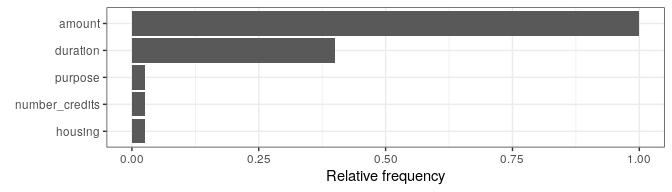The parallel plot connects the (scaled) feature values of each counterfactual and highlights x_interest in blue. We specify feature_names to order the features according to their frequency of changes. cfactuals$plot_parallel(feature_names = names(
cfactuals$get_freq_of_feature_changes()), digits_min_max = 2L)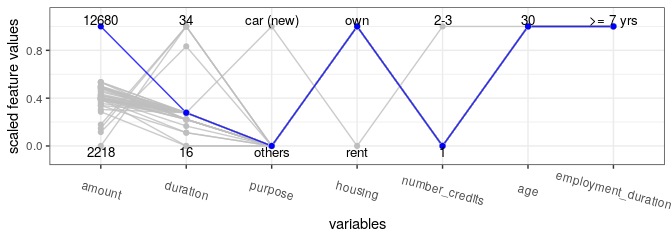In the following surface plot, the white dot represents x_interest. All counterfactuals that differ from x_interest only in the selected features are displayed as black dots. The tick marks next to the axes indicate the marginal distribution of the counterfactuals. cfactuals$plot_surface(feature_names = c("duration", "amount"))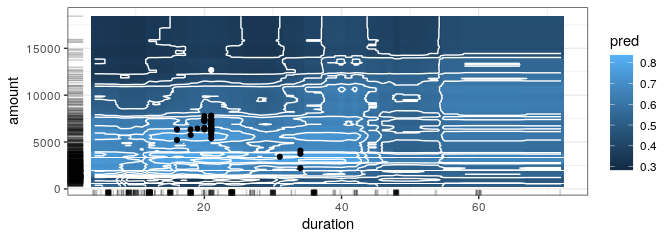### MOC diagnostics

Additional diagnostic tools for MOC are available as part of the MOCClassif and MOCRegr class. For example, the hypervolume indicator (Zitzler and Thiele 1998) given a reference point (that represents the maximal values of the objectives) could be computed. The evolution of the hypervolume indicator can be plotted together with the evolution of mean and minimum objective values using the plot_statistics() method.

moc_classif$plot_statistics(centered_obj = TRUE) []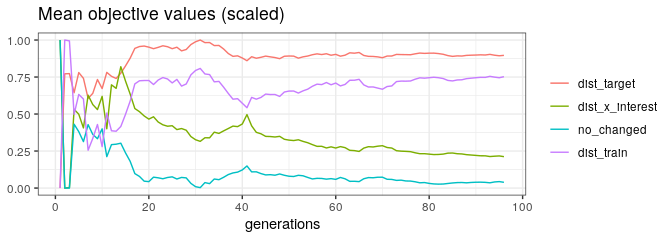[]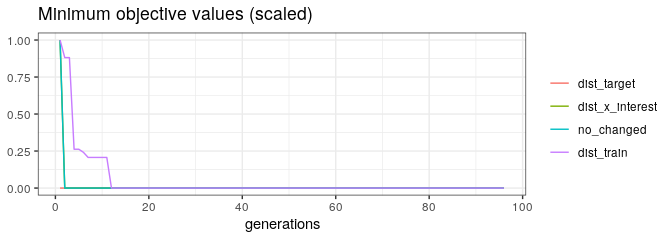[]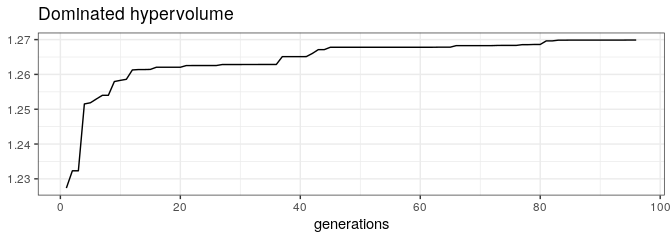Ideally, one would like the mean value of each objective to decrease over the generations, leading to an increase of the hypervolume. We could visualize the objective values of the emerging candidates throughout the generations via the plot_search method for pairs of objectives. moc_classif$plot_search(objectives = c("dist_train", "dist_target"))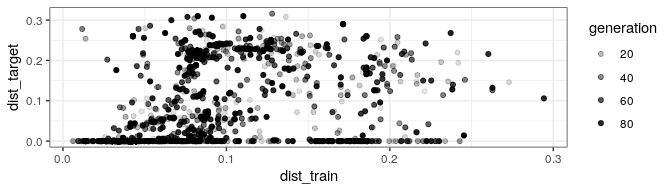moc_classif$plot_search(objectives = c("dist_x_interest", "dist_train"))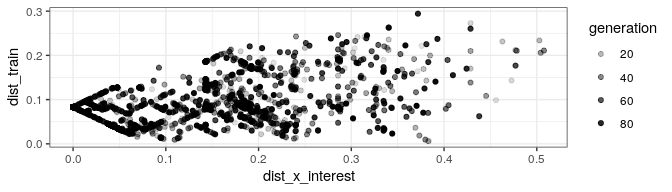## Regression Tasks Finding counterfactuals for regression models is analogous to classification models. In this example, we use NICE (Brughmans et al. (2002)) to search for counterfactuals for plasma . Brughmans et al. introduced NICE only for the classification setting but for this package the method was extended to also work for regression tasks by allowing prediction functions to return real-valued outcomes instead of classification scores. ### Data: Plasma Retinol As training data, we use the plasma dataset from the gamlss.data package. The dataset contains 315 observations with 13 features and the (continuous) target variable retplasma, the plasma retinol concentration in ng/ml. The plasma retinol concentration is interesting because low concentrations are associated with an increased risk for some types of cancer (Harrell 2022). data(plasma, package = "gamlss.data") Variable Description age Age (years) sex Sex (1 = male, 2 = female) smokstat Smoking status (1 = never, 2 = former, 3 = current smoker) bmi Body mass index (weight/(height^2)) vituse Vitamin use (1 = yes, fairly often, 2 = yes, not often, 3 = no) calories Number of calories consumed per day fat Grams of fat consumed per day fiber Grams of fiber consumed per day alcohol Number of alcoholic drinks consumed per week cholesterol Cholesterol consumed (mg per day) betadiet Dietary beta-carotene consumed (mcg per day) retdiet Dietary retinol consumed (mcg per day) betaplasma Plasma beta-carotene (ng/ml) retplasma Plasma retinol (ng/ml) ### Fitting a model First, we train a model to predict plasma_retinol, again omitting x_interest from the training data. This time we use a regression tree trained with the mlr3 and rpart package. tsk = mlr3::TaskRegr$new(id = "plasma", backend = plasma[-100L,], target = "retplasma")
tree = lrn("regr.rpart")
model = tree$train(tsk) ### Setting up an iml::Predictor() object Then, we initialize an iml::Predictor object. predictor = iml::Predictor$new(model, data = plasma, y = "retplasma")
x_interest = plasma[100L, ]
predictor$predict(x_interest) #> predict.model..newdata...newdata. #> 1 342.9231 For x_interest, the model predicts a plasma concentration of 342.92. ### Find counterfactuals Since we want to apply NICE to a regression model, we initialize a NICERegr object. For regression models, we define a correctly predicted datapoint when its prediction is less than a user-specified value away. Here we allow for a deviation of margin_correct = 0.5. In this example, we aim for proximal counterfactuals in additional to sparse ones, such that we set optimization = "proximity". nice_regr = NICERegr$new(predictor, optimization = "proximity",
margin_correct = 0.5, return_multiple = FALSE)

Then, we use the find_counterfactuals() method to find counterfactuals for x_interest with a predicted plasma concentration in the interval [500, Inf].

cfactuals = nice_regr$find_counterfactuals(x_interest, desired_outcome = c(500, Inf)) ### The counterfactuals object As a result, we obtain a Counterfactuals object, just like for the classification task. cfactuals #> 1 Counterfactual(s) #> #> Desired outcome range: [500, Inf] #> #> Head: #> age sex smokstat bmi vituse calories fat fiber alcohol cholesterol betadiet retdiet betaplasma #> 1: 46 1 3 35.25969 3 2667.5 131.6 10.1 0 550.5 1210 1291 218 To inspect the counterfactual, we can use the same tools as before. For example, in the surface plot, we see that increasing betaplasma helps while changing the age alone has no impact on the prediction. cfactuals$plot_surface(feature_names = c("betaplasma", "age"), grid_size = 200)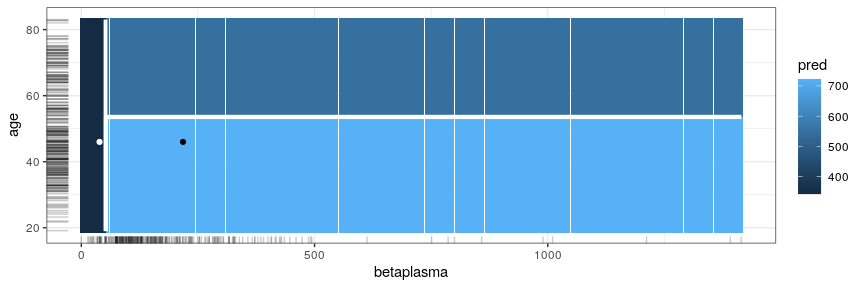### User-defined distance function

At the beginning, NICE calculates the distance of x_interest to each of the training samples. By default, Gower’s distance measures this but users could also specify their own distance functions in the distance_function argument. For example, the Gower distance can be replaces by the L_0 norm.

l0_norm = function(x, y, data) {
res = matrix(NA, nrow = nrow(x), ncol = nrow(y))
for (i in seq_len(nrow(x))) {
for (j in seq_len(nrow(y))) {
res[i, j] = sum(x[i,] != y[j,])
}
}
res
}

A short example illustrates the functionality of l0_norm().

xt = data.table::data.table(a = c(0.5), b = c("a"))
yt = data.table::data.table(a = c(0.5, 3.2, 0.1), b = c("a", "b", "a"))
l0_norm(xt, yt, data = NULL)
#>      [,1] [,2] [,3]
#> [1,]    0    2    1

Replacing the distance function is fairly easy:

nice_regr = NICERegr$new(predictor, optimization = "proximity", margin_correct = 0.5, return_multiple = FALSE, distance_function = l0_norm) cfactuals = nice_regr$find_counterfactuals(x_interest, desired_outcome = c(500, 1000))
cfactuals
#> 1 Counterfactual(s)
#>
#> Desired outcome range: [500, 1000]
#>
#> 1:  46   1        3 35.25969      3   2667.5 131.6  10.1       0       550.5     1210    1291        218`